Next: About this document ... Up: lab_template Previous: lab_template

Subsections

# Assigning labels, expressions and functions

## Introduction

The purpose of this lab is to introduce you to the basics needed in any Maple lab. The main topics include: assigning labels, defining expressions, defining functions and plotting.

## Entering an expression

Expressions such ascan be entered in a similar way to variable assignment. Choose a variable name to represent the expression and assign the expression to the variable as follows.
> j := x^3+3*x^2-x+1;


## Entering a function

Suppose you want to enter the same expression stored in expr above, but as a function of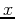. In Maple, you would type
> f := x-> x^3+3*x^2-x+1;

Below is how NOT to enter a function:
> f(x) := x^3+3*x^2-x+1;

The difference between expressions and functions are first the obvious, that expressions do not have to satisfy the definition of a function in the sense that for each input, there is a unique value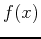. A function may be defined as an expression, but not all expressions can be defined as functions. The differences in Maple are numerous as you will see below when we evaluate the expression or function for a given value as well as when using the plot command.

## Evaluating functions and expressions

In order to evaluate an expression at a given value of, you must use the subs or eval command. For example, if we wanted to evaluate the expression expr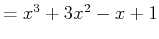at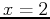, the example below show how this can be done
> subs(x=2,j);
> eval(j,x=2);
> r:=sin(theta) + 8*theta^2;
> subs(theta=1/2*Pi,r);

In the subs command, the first argument tells Maple what you would like to substitute in for. The second argument tells Maple what expression you are substituting into. Note the difference in outputs when a whole number or fraction is entered compared to a decimal.
>g:=2*x/3;
>subs(x=4,g);
>eval(g,x=1/2);
>subs(x=4.0,g);

In Maple, functions are much easier to evaluate than expressions. In order to evaluate the function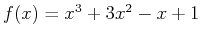at, simply type
> f(2);

Here are a few more examples of evaluating functions.
> f(a+h);
> f(Pi);
> evalf(f(Pi));

Note the use of the evalf command in the last example above. This command is used when we want Maple to output the answer in decimal form. If this command is not used, the output to your Maple commands will be calculated analytically, where as the evalf command forces Maple to calculate the answers numerically. The evalf command has one essential argument, however a second argument can be added in order to tell how many digits we want to be in the answer. The example below evaluatesusing 10 digits, not 10 decimal places.
> evalf(Pi,10);


## Plotting

The following example shows how the Maple plot command is used to plot the previously entered functionand expressions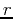.
> plot(f(x),x=-5..2);
> plot(r,theta=-2*Pi..2*Pi);

The plot command above has two essential arguments. The first argument is the function(s) or expression(s) that you want to plot. The function or expression can be typed in before the plot command or you can simply type it in as the first argument. The second argument is the range of numbers for the-axis. The plot command allows you to add additional optional arguments as well. For instance, you may want to also restrict the y-axis range or add a title to your plot.
> plot(x^2,x=-2..2,y=-5..5,title="My First Plot");

This particular command allows you to add arguments, but if you were to leave off one of the essential arguments, you will get an error message. You can also plot more than one function or expression on the same graph by enclosing them in square brackets [ ] and separating them by commas. For example, we can plotandon the same graph.
> f := x-> x^2-2;
> g := x-> -x+2;
> plot([f(x),g(x)],x=-4..4,color=["DarkOrchid","Gold"]);
>?plot,colornames


## Solving Equations

To find the intersection of the parabola and line defined above asand, use Maple's solve or fsolve commands as in the examples below. It is important to assign a variable to the solutions of the solve command for ease of evaluating the correspondingcoordinate for eachcoordinate.
> a:=solve(f(x)=g(x),x);
> f(a);
> f(a);
> b:=fsolve(f(x)=g(x),x=-5..0);
> c:=fsolve(f(x)=g(x),x=0..5);
> f(a);
> f(b);


## Exercises

1. Enteras an expression.
A)
Evaluate this expression at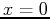.
B)
Evaluate this expression at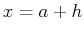.
C)
Evaluate this expression at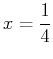.
D)
Evaluate this expression at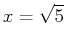and make sure your output is in decimal form.
E)
Plot this expression over therange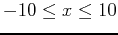.
2. Repeat all parts of exercise 1 by first definingas a function.
3. Define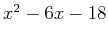and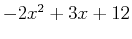as functions. Plot them both on the same graph. Find the intersection points by using Maple's solve command and label the output with a variable. Use the variable to find thevalues. State intersection points in a sentence in text format of Maple.
4. Define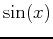and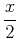as functions. Plot them both on the same graph. Find the intersection points by using Maple's fsolve command and label the output with a variable. Use the variable to find thevalues. State intersection points in a sentence in text format of Maple.Next: About this document ... Up: lab_template Previous: lab_template
Dina J. Solitro-Rassias
2017-08-28